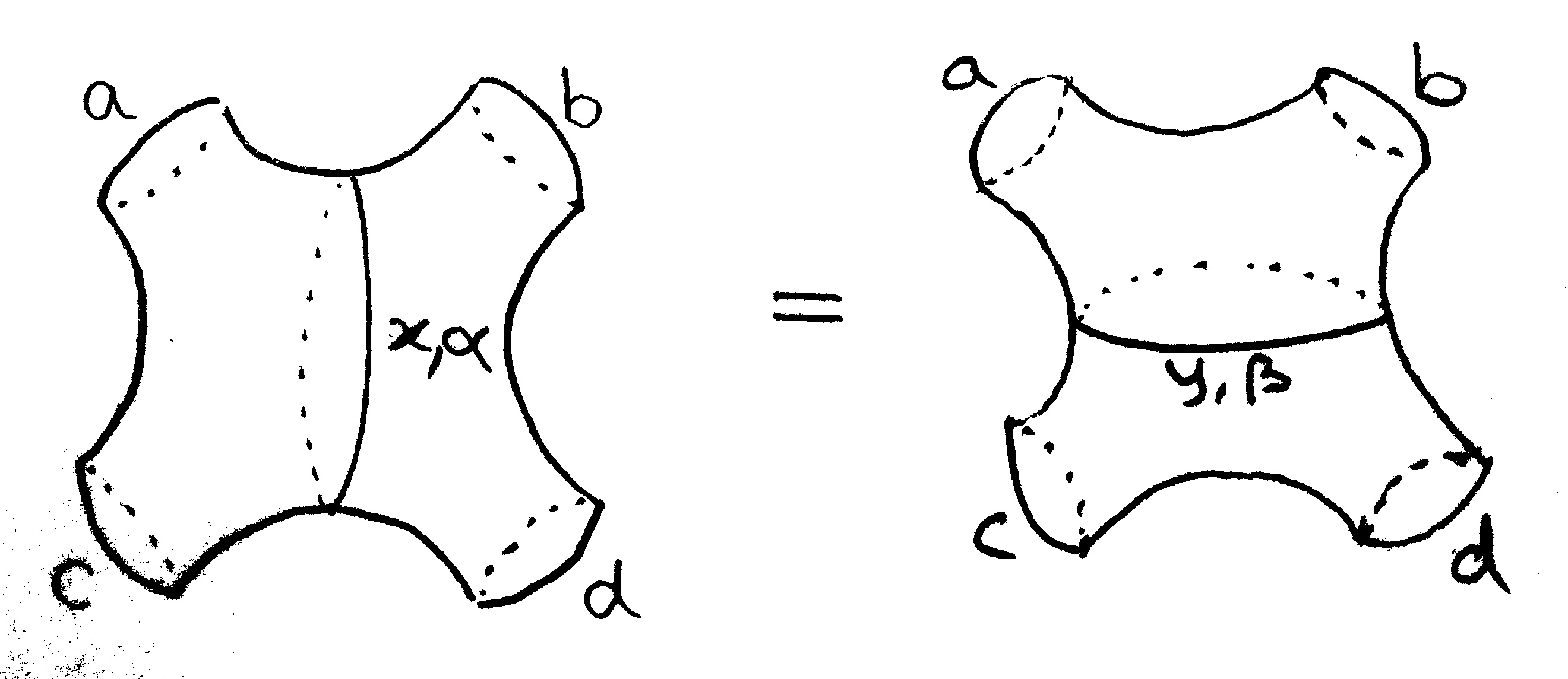# Change of coordinates for Teichmüller space of the 4-holed sphere

The diagram below indicates 2 ways to use Fenchel-Nielsen coordinates to parameterize the Teichmüller space of conformal structures on the 4-holed sphere with totally-geodesic boundary, corresponding to the two pants decompositions, as indicated by this diagram:Here $a,b,c,d,x,y$ are lengths of the indicated geodesics in a hyperbolic instance of the conformal structure, and $\alpha,\beta$ are the twisting parameters for the gluing.

What is the coordinate transformation implied by these decompositions? That is, what is the formula for $y$ and $\beta$ as a function of $a,b,c,d,x,\alpha$?

• Just to be precise, there are infinitely many ways to give FN coordinates for the four-holed torus, one for every essential non-peripheral simple closed curve. – Sam Nead Mar 2 '14 at 14:32
• Indeed, thanks for that point, I will edit the question to clarify this. – Jamie Vicary Mar 2 '14 at 14:36

See the paper "Effects of a change of pants decompositions on their Fenchel-Nielsen coordinates" by Takayuki Okai, published in Kobe J. Math.

You can also find a version where some of the boundary components are cusps in the paper "The behaviour of Fenchel-Nielsen distance under a change of pants decomposition" by Alessandrini, Liu, Papadopoulos, and Su, published in Comm. Anal. Geom. and also available on the ArXiv.

Added by @JamieVicary: here are the equations as given in the Alessandrini, Liu and Papadopoulos paper in the notation of this question, with $a'=\text{cosh}(a/2)$, $b'=\text{cosh}(b/2)$, $c'=\text{cosh}(c/2)$, $d'=\text{cosh}(d/2)$, $x'=\text{cosh}(x/2)$, $y'=\text{cosh}(y/2)$, $\alpha' = \text{cosh}(\alpha)$ and $\beta' = \text{cosh}(\beta)$:

\begin{align} x'&=y' ^{-2} ( a'c'+d'b' + y'(a'd' + c'b') + \beta'(y'^2 + 2 a'b'y' + a'^2 + b'^2 - 1] ^{1/2}(y'^2 + 2 c'd'y' + c'^2 + d'^2)^{1/2}) \\ \alpha'&=(a'^2 + c'^2 + 2 a' c' x' + x'^2-1) ^{-1/2} ( d'^2 + b'^2 + 2 d' b' x' + x'^2 - 1) ^{-1/2} ((x'^2 - 1)y' - a'b' - c'd' - x'(a'd' + c'b')) \end{align}

• Hasn't this all been done earlier by Penner, with his Lambda lengths? – Igor Rivin Mar 2 '14 at 17:23
• @Igor - As far as I understand them, Penner's lambda lengths require that the surface have punctures, and also a choice of ideal triangulation (instead of a pants decomposition). – Sam Nead Feb 9 '16 at 19:49
• I think that if you allow spun triangulations (or whatever they are called), everything works fine... – Igor Rivin Feb 9 '16 at 19:58
• From a pair of pants decomposition of the surface, if you choose an orientation on each pants curve you get a natural spun triangulation. There are coordinates associated to spun triangulations in general. These are close to the Fenchel-Nielsen coordinates, but not quite the same. But then changing the spun triangulation to do the necessary pants move is not obvious. (Which is all to say that Igor's idea is not crazy, but is complicated to work out.) – Dylan Thurston May 9 '17 at 10:20
• Do you think these transformations can be put in a cluster-algebra form? – giulio bullsaver Jul 14 '18 at 0:09